## Introduction

The R-package RIbench implements a benchmarking suite for the standardized evaluation of indirect methods for reference interval estimation. A manuscript describing the concept and a first application was recently published (Ammer et al., Clin Chem 2022). The benchmark suite contains simulated test sets of ten biomarkers mimicking routine measurements of a mixed distribution of non-pathological and pathological values. The non-pathological distributions are representative of the most common distribution types observed in laboratory practice: normal, skewed, heavily skewed, skewed-and-shifted. As indirect methods usually operate on real-world data (RWD), pathological distributions with varying location, fraction, and extent of overlap with the non-pathological distribution, are added. Further, the sample size is varied to get a broad variety of test sets. Overall, the benchmark suite contains 5,760 test sets, 576 per biomarker. The R-package offers various convenience functions to generate the datasets, run the estimations using an existing or novel indirect method, as well as to evaluate the results and generate plots.

## Process Overview

The three main functions and steps to evaluate one’s own algorithm and reproduce the evaluation and the computation of the benchmark score as shown in Ammer et al., 2022 are the following:

# load RIbench package
library(RIbench)
# set directory from where a ./Data folder will be generated
workingDir <- tempdir()

# generate all test sets
generateBiomarkerTestSets(workingDir = workingDir)

# evaluate all test sets using existing or new indirect method ('myOwnAlgo')
#    with pre-specified R-function ('estimateModel') (provided by the user)
evaluateBiomarkerTestSets(workingDir = workingDir, algoName = 'myOwnAlgo', algoFunction = 'estimateModel', libs = c('myOwnAlgo'))

# evaluate all results, create plots and compute the benchmark score
benchmarkScore <- evaluateAlgorithmResults(workingDir = workingDir, algoNames = "myOwnAlgo")

First, the benchmark test sets are generated and saved into a specified working directory (generateBiomarkerTestSets()). Following that, either an existing or a new algorithm can be called to estimate RIs for each of these simulated test sets (evaluateBiomarkerTestSets()). Here, a user-defined wrapper function is required to call the existing or new algorithm and return the result in the required format. Results are then evaluated using evaluateAlgorithmResults() to generate graphical representations and compute the benchmark score.

More details for the main functions are provided in the following chapters.

## Generate Biomarker Test Sets

The R-package RIbench contains a table with pre-defined parameters enabling the generation of a standardized benchmark dataset with 5,760 individual test sets. These simulated test sets mimic routine measurements of ten biomarkers observed in laboratory practice. The biomarkers were chosen to represent the most common distribution types occurring in laboratory practice:

• (approximately) normal: Hemoglobin (Hb), Calcium (Ca), Free Thyroxine (FT4)
• skewed: Aspartate transaminase (AST), Lactate (LACT), Gamma-Glutamyltransferase (GGT)
• heavily skewed: Thyroid-stimulating hormone (TSH), Immunoglobulin E (IgE), C-reactive protein (CRP)
• skewed-and-shifted: Lactate dehydrogenase (LDH)

The non-pathological distributions of these biomarkers are simulated using one- or two-parameter Box-Cox transformed normal distributions, and are defined by the parameters nonp_lambda, nonp_mu, nonp_sigma, and nonp_shift. To simulate RWD, pathological distributions are added to the left and the right side of the non-pathological distribution. These are simulated using normal distributions and are defined by left_mu and left_sigma, right_mu and right_sigma respectively. To ensure a broad variety of test sets, the following parameters are varied:

• sample size (N)
• overall pathological fraction (fractionPathol)
• for each biomarker, two different scenarios are defined by varying the following parameters:
• ratio between fraction of “left” and “right” pathological fraction (left_ratio, right_ratio)
• location (mu) and width (sigma) of pathological distributions
• overlap between pathological and non-pathological distributions (overlapPatholLeft and overlapPatholRight)

To simulate inconsistencies in RWD, we added a background “noise” distribution based on uniform distribution defined by (bg_min and bg_max) with a fixed fraction of 0.1% (bg_fraction).

To take a look at the test set definitions, you can load the overview table or to get a comprehensive overview, see Ammer et al., 2022, Table 1.

# load RIbench package and load testset definition
library(RIbench)
str(testsets)
## 'data.frame':    5760 obs. of  38 variables:
##  $Index : int 1 2 3 4 5 6 7 8 9 10 ... ##$ Analyte           : chr  "Hb" "Hb" "Hb" "Hb" ...
##  $startSeed : int 123 123 123 123 123 123 123 123 123 123 ... ##$ Distribution      : chr  "normal" "normal" "normal" "normal" ...
##  $N : num 1000 1000 1000 1000 1000 1000 1000 1000 1000 1000 ... ##$ Scenario          : chr  "Sc1" "Sc2" "Sc1" "Sc2" ...
##  $fractionPathol : num 0 0 0.05 0.05 0.1 0.1 0.2 0.2 0.3 0.3 ... ##$ overlapPatholLeft : chr  "low" "low" "low" "low" ...
##  $overlapPatholRight: chr "low" "low" "low" "low" ... ##$ nonp_lambda       : num  1 1 1 1 1 1 1 1 1 1 ...
##  $nonp_mu : num 13 13 13 13 13 13 13 13 13 13 ... ##$ nonp_sigma        : num  1.02 1.02 1.02 1.02 1.02 ...
##  $nonp_shift : int 0 0 0 0 0 0 0 0 0 0 ... ##$ bg_min            : int  0 0 0 0 0 0 0 0 0 0 ...
##  $bg_max : int 160 160 160 160 160 160 160 160 160 160 ... ##$ bg_fraction       : num  0.001 0.001 0.001 0.001 0.001 0.001 0.001 0.001 0.001 0.001 ...
##  $left_xOv : int 5 2 5 2 5 2 5 2 5 2 ... ##$ left_mu           : num  10.18 8.94 10.18 8.94 10.18 ...
##  $left_sigma : num 1.03 1.6 1.03 1.6 1.03 1.6 1.03 1.6 1.03 1.6 ... ##$ right_xOv         : int  5 2 5 2 5 2 5 2 5 2 ...
##  $right_mu : num 17.8 18.1 17.8 18.1 17.8 ... ##$ right_sigma       : num  1.03 1.1 1.03 1.1 1.03 1.1 1.03 1.1 1.03 1.1 ...
##  $left_ratio : int 1 3 1 3 1 3 1 3 1 3 ... ##$ right_ratio       : int  1 1 1 1 1 1 1 1 1 1 ...
##  $ratio_sum : int 2 4 2 4 2 4 2 4 2 4 ... ##$ decimals          : int  1 1 1 1 1 1 1 1 1 1 ...
##  $GT_LRL : num 12 12 12 12 12 12 12 12 12 12 ... ##$ GT_URL            : num  16 16 16 16 16 16 16 16 16 16 ...
##  $Unit : chr "mg/dL" "mg/dL" "mg/dL" "mg/dL" ... ##$ left_Ov           : num  0 0 6.23 7.08 9.97 ...
##  $left_OvFreq : num 0 0 0.455 0.4 1.345 ... ##$ right_Ov          : num  0 0 4.99 5.44 8.48 ...
##  $right_OvFreq : num 0 0 0.752 0.379 1.615 ... ##$ Ov                : num  0 0 11.2 12.5 18.4 ...
##  $OvFreq : num 0 0 1.207 0.779 2.96 ... ##$ RuntimeSet        : int  0 0 0 0 0 1 0 0 0 0 ...
##  $HashCode : chr "a80c00da90a1958b01ca1e63bb0de66a" "d6a83f9330cab1d5c10d6f0e6c182b66" "42d27ccbe3f5c59343a16a80fcd714e8" "f1a0115fe6d2a8bdb00c245efd0e7fb2" ... ##$ HashCodeNotRounded: chr  "18e426882acd757e7a812eb740ae0715" "6ab61d0a5f57bcb4e401d3c8ec45d1f2" "9f24d9cc8f88193b5a9fa8bd4827c829" "e2f9911f94be2074f8b895f10801f2de" ...

RIbench offers a convenience function to generate all test sets using generateBiomarkerTestSets(). Here, first a directory structure is set up and all defined biomarker test sets (or a specified subset thereof) are generated and saved as .csv file. Using pre-specified initialization seeds for the random number generator ensures reproducibility and objective comparison of results obtained by different users. The function can take following arguments:

• workingDir … (character) specifying the working directory from which a ./Data directory will be generated
• subset … (character, numeric, or data.frame) to specify for which subset the algorithm should be executed.
• character options:
• ‘all’ (default) for all test sets
• distribution type: ‘normal’, ‘skewed’, ‘heavilySkewed’, ‘shifted’
• a biomarker: ‘Hb’, ‘Ca’, ‘FT4’, ‘AST’, ‘LACT’, ‘GGT’,‘TSH’, ‘IgE’, ‘CRP’, ‘LDH’)
• ‘runtime’ for runtime analysis subset
• numeric option: number of test sets per biomarker, e.g. 10
• data.frame: customized subset of table with test set specification
• rounding … (logical) indicating whether decimal places stated in test set definitions should be applied (default, TRUE), if FALSE, data will be rounded to 5 decimal places to mimic not rounded data
# set directory from where a ./Data folder will be generated
workingDir <- tempdir()
print(workingDir)
# generate all test sets
generateBiomarkerTestSets(workingDir = workingDir)

## Evaluate Biomarker Test Sets

### Inclusion of New Indirect Method

#### Indirect Method that Estimates the Parameters of a (Shifted) Box-Cox Transformed Normal Distribution

Provide a wrapper function for your own method (e.g. estimateModel()). This function should have an input for the dataset (Data), and inputs for any additional parameters required for the algorithm (). It should return an RWDRI object with the estimated model parameters (lambda, mu, sigma, shift). The function should then be saved into an R-source file, MyAlgoWrapper.R.

# load R-package with the indirect method to be investigated
library(myOwnAlgo)

estimateModel <- function(Data = NULL, ... ){

# PLACEHOLDER: insert your own function for the estimation of a (shifted) Box-Cox transformed normal distribution here

# Initialize an RWDRI object with the estimated model parameters (lambda, mu, sigma, shift) and return it
obj         <- list()
obj$Lambda <- lambda # power parameter lambda, only if Box-Cox transformation is integrated into your method. # For normal distribution set to 1 and subtract 1 from the estimated mean. obj$Mu      <- mu       # mean
obj$Sigma <- sigma # standard deviation obj$Shift   <- shift    # shift, only if 2-parameter Box-Cox transformation is integrated into your method,
# otherwise set to 0.
obj$Method <- "myOwnAlgo" class(obj) <- "RWDRI" return(obj) } #### Indirect Method that Estimates Reference Intervals Directly Provide a wrapper function for your own method (e.g. estimateRIs()). This function should have an input for the dataset (Data), an input for the specified percentiles (percentiles) used as RIs and inputs for any additional parameters required for the algorithm (). It should return the estimates for the specified percentiles in the format shown below. The function should then again be saved into an R-source file, e.g. MyAlgoWrapper.R. # load R-package with the indirect method to be investigated library(myOwnAlgo) estimateRIs <- function(Data = NULL, percentiles = c(0.025,0.975), ... ){ # PLACEHOLDER: insert your own function to estimate reference intervals here # Initialize a data frame with the specified percentiles and fill the PointEst column with the corresponding # estimates obtained by your own method (RIResultMyOwnAlgo) RIResult <- data.frame(Percentile = percentiles, PointEst = NA) RIResult$PointEst <- RIResultMyOwnAlgo

return(RIResult)
}

### Evaluate Biomarker Test Sets using Indirect Method

To estimate RIs for all test sets in the benchmark suite, call evaluateBiomarkerTestSest() with the name of the algorithm to test (algoName, e.g. myOwnAlgo), the name of the wrapper function (algoFunction, e.g. estimateModel), a vector with the required libraries (libs, e.g. myOwnAlgo), a list with the source files containing the wrapper function and all other needed functions (sourceFiles, e.g. MyAlgoWrapper.R) and a list with the required parameters for the algorithm (params). If the method additionaly requires the number of decimal points as input, set requireDecimals to TRUE. If the method directly estimates reference intervals, set requirePercentiles to TRUE.

Overall, the evaluateBiomarkerTestSets() function takes the following arguments:

• workingDir … (character) specifying the working directory: Results will be stored in workingDir/Results/algoName/biomarker and data will be used from workingDir/Data/biomarker
• algoName … (character) specifying the algorithm that should be called
• algoFunction … (character) specifying the algorithm that should be called
• libs … (list) containing all libraries needed for executing the algorithm
• sourceFiles … (list) containing all source files needed for executing the algorithm, e.g. file with defined wrapper function
• params … (list) with additional parameters needed for calling algoFunction
• requireDecimals … (logical) indicating whether algorithm needs the number of decimal places (TRUE) or not (FALSE, default)
• requirePercentiles … (logical) indicating whether only percentiles and no model is estimated
• subset … (character, numeric, or data.frame) to specify for which subset the algorithm should be executed.
• character options:
• ‘all’ (default) for all test sets
• distribution type: ‘normal’, ‘skewed’, ‘heavilySkewed’, ‘shifted’
• a biomarker: ‘Hb’, ‘Ca’, ‘FT4’, ‘AST’, ‘LACT’, ‘GGT’,‘TSH’, ‘IgE’, ‘CRP’, ‘LDH’
• ‘runtime’ for runtime analysis subset
• numeric option: number of test sets per biomarker, e.g. 10
• data.frame: customized subset of table with test set specification
• timeLimit … (integer) specifying the maximum amount of time in seconds allowed to execute one single estimation (default: 14400 sec (4h))
# The evaluation of all test sets can take several hours or longer depending on the computation time of the algorithm

evaluateBiomarkerTestSets(workingDir = workingDir, algoName = 'myOwnAlgo', algoFunction = 'estimateModel',
libs = c('myOwnAlgo'), sourceFiles = list("MyAlgoWrapper.R"),
requireDecimals = FALSE, requirePercentiles = FALSE,
subset ='all', timeLimit = 14400)

To ensure a robust flow of computations, different ‘fail-safe’ features are included: First, a time limit per calculation is implemented (timeLimit), with a default of four hours to facilitate a timely execution of all computations. Second, if an algorithm fails or crashes the encapsulated R session, this failure status is documented. Third, the calculation progress is saved, and can be restored if the evaluation is interrupted by external factors (e.g. an unintended restart of the computer).

### Custom Options

#### Definition of Subsets

Using the subset parameter, different customized subgroups of the whole benchmark suite can be evaluated with the indirect method.

First, the methods can be applied to the test sets of only one biomarker, e.g. Calcium, by setting the subset parameter to the abbreviation for each biomarker stated in the function documentation, e.g. for Calcium use subset=‘Ca’.

# Exemplary evaluation for only 'Calcium' test sets.
progress <- evaluateBiomarkerTestSets(workingDir = workingDir, algoName = 'myOwnAlgo', algoFunction = 'estimateModel',
libs = c('myOwnAlgo'), subset = "Ca")

Second, the indirect methods can be applied to only one distribution type, e.g. all test sets following a skewed distribution, by setting subset to this distribution type, e.g. subset = ‘skewed’”.

# Exemplary evaluation for only a subset testsets that follow a skewed distribution.
progress <- evaluateBiomarkerTestSets(workingDir = workingDir, algoName = 'myOwnAlgo', algoFunction = 'estimateModel',
libs = c('myOwnAlgo'), subset = "skewed")

Third, only a restricted number of test sets per biomarker can be evaluated by the indirect method, by setting the subset parameter to an integer between 0 and 576, e.g. subset = 3.

# Exemplary evaluation for a subset of 3 testsets per biomarker.
progress <- evaluateBiomarkerTestSets(workingDir = workingDir, algoName = 'myOwnAlgo', algoFunction = 'estimateModel',
libs = c('myOwnAlgo'), subset = 3)

Forth, a customized subset can be defined using the provided test set specification table. Thus, only part of the benchmark suite with certain characteristics can be evaluated, e.g. all test sets with a pathological fraction <= 30%. Here, the subset parameter shall be set to the customized subset of the test set definition data frame, e.g. subset = testsets[testsets$fractionPathol <= 0.3,]. testsets <- loadTestsetDefinition() # Exemplary evaluation for a customized subset with all test sets that have a pathological fraction <= 30%. progress <- evaluateBiomarkerTestSets(workingDir = workingDir, algoName = 'myOwnAlgo', algoFunction = 'estimateModel', libs = c('myOwnAlgo'), subset = testsets[testsets$fractionPathol <= 0.3,] )

If the customized model estimation function requires additional parameters, these can be specified by the params argument. For example, if an algorithm offers the option to try to estimate a 2-parameter Box-Cox transformation (e.g. like refineR) to better model skewed and shifted distribution (e.g. as simulated in the LDH case), you can use this option by setting the required parameter in the params arugment, e.g. params = list(“model = ‘2pBoxCox’”):

# Define wrapper function with the additional 'model' argument and save to script e.g. called 'Test_RIEst_2pBoxCox.R'

estimateModelDec <- function(Data = NULL, model = NULL, ... ){

# PLACEHOLDER: insert your own function for estimation of a (shifted) Box-Cox transformed normal distribution here

# Initialize an RWDRI object with the estimated model parameters (lambda, mu, sigma, shift) and return it
obj         <- list()
obj$Lambda <- lambda # power parameter lambda, only if Box-Cox transformation is integrated into your method. # For normal distribution set to 1 and subtract 1 from the estimated mean. obj$Mu      <- mu       # mean
obj$Sigma <- sigma # standard deviation obj$Shift   <- shift    # shift, only if 2-parameter Box-Cox transformation is integrated into your method,
# otherwise set to 0.
obj$Method <- "myOwnAlgo" class(obj) <- "RWDRI" return(obj) } progress <- evaluateBiomarkerTestSets(workingDir = workingDir, algoName = 'myOwnAlgo', algoFunction = 'estimateModel', libs = c('myOwnAlgo'), sourceFiles = list("Test_RIEst_2pBoxCox"), params = list("model='2pBoxCox'")) If an indirect method requires the number of decimal places for the estimation of RIs, like kosmic, TMC, or TML, the requireDecimals parameter need to be set to TRUE. Further, the wrapper function should have an argument called decimals that will be used to forward the specified decimal points to the algorithm. # Define wrapper function and save to script e.g. called 'Test_RIEst_dec.R' estimateModelDec <- function(Data = NULL, decimals = NULL, ... ){ # PLACEHOLDER: insert your own function for estimation of a (shifted) Box-Cox transformed normal distribution here # Initialize an RWDRI object with the estimated model parameters (lambda, mu, sigma, shift) and return it obj <- list() obj$Lambda  <- lambda   # power parameter lambda, only if Box-Cox transformation is integrated into your method.
# For normal distribution set to 1 and subtract 1 from the estimated mean.
obj$Mu <- mu # mean obj$Sigma   <- sigma    # standard deviation
obj$Shift <- shift # shift, only if 2-parameter Box-Cox transformation is integrated into your method, # otherwise set to 0. obj$Method  <- "myOwnAlgo"
class(obj)  <- "RWDRI"

return(obj)
}

evaluateBiomarkerTestSets(workingDir = workingDir, algoName = 'myOwnAlgo', algoFunction = 'estimateModelDec',
libs = c('myOwnAlgo'), sourceFiles = "Test_RIEst_dec.R",
requireDecimals = TRUE)

To evaluate a function that directly estimates reference intervals / percentiles, set the requirePercentiles parameter to TRUE. The provided wrapper function should have an additional argument called percentiles to forward the specified percentiles and should return the estimated percentiles in a data frame as shown.

# save e.g. the following function into a script called "Test_RIEst.R"

# load R-package with the indirect method to be investigated
library(myOwnAlgo)

# function requires an argument called percentiles to use that for the direct estimation of the specified percentiles
estimateRIs <- function(Data = NULL, percentiles = c(0.025,0.975), ... ){

# estimate reference intervals with custom defined function
RIResultMyOwnAlgo <- findPerc(Data)

# save estimations into required format
RIResult          <- data.frame(Percentile = percentiles, PointEst = RIResultMyOwnAlgo)

return(RIResult)
}

# set requirePercentile to TRUE and specify the file that contains the R code for the wrapper function
#    for the direct estimation of reference intervals / percentiles
evaluateBiomarkerTestSets(workingDir = workingDir, algoName = "myOwnAlgo", algoFunction = "estimateRIs",
libs = "myOwnAlgo", sourceFiles = "Test_RIEst.R",
requirePercentiles = TRUE) 

## Evaluate Algorithm Results

### Default Evaluation

To evaluate all testsets and generate all result plots featured in Ammer et al., 2022, and to calculate the benchmark score, a convenience function evaluateAlgorithmResults() is provided. The function takes the following arguments:

• workingDir …(character) specifying the working directory: Plots will be stored in workingDir/evalFolder and results will be used from workingDir/Results/algoName/biomarker
• algoNames …(character) vector specifying all algorithms that should be part of the evaluation
• subset … (character, numeric, or data.frame) to specify for which subset the algorithm should be executed.
• character options:
• ‘all’ (default) for all test sets
• distribution type: ‘normal’, ‘skewed’, ‘heavilySkewed’, ‘shifted’
• a biomarker: ‘Hb’, ‘Ca’, ‘FT4’, ‘AST’, ‘LACT’, ‘GGT’,‘TSH’, ‘IgE’, ‘CRP’, ‘LDH’
• ‘runtime’ for runtime analysis subset
• numeric option: number of test sets per biomarker, e.g. 10
• data.frame: customized subset of table with test set specification
• cutoffZ …(integer) specifying if and if so which cutoff for the absolute z-score deviation should be used to classify results as implausible and exclude them from the overall benchmark score (default: 5)
• evalFolder …(character) specifying the name of the ouptut directory, Plots will be stored in workingDir/evalFolder, default: ‘Evaluation’
• withDirect …(logical) indicating whether the direct method should be simulated for comparison (default:TRUE)
• withMean …(logical) indicating whether the mean should be plotted as well (default: TRUE)
• outline …(logical) indicating whether outliers should be drawn (TRUE, default), or not (FALSE)
• errorParam …(character) specifying for which error parameter the data frame should be generated, choose between absolute z-score deviation (“zzDevAbs_Ov”), absolute percentage error (“AbsPercError_Ov”), and absolute error (“AbsError_Ov”)
• cols …(character) vector specifying the colors used for the different algorithms
• … additional arguments to be passed to the method,e.g. “truncNormal” (logical) vector specifying if a normal distribution truncated at zero shall be assumed, can be either TRUE/FALSE or a vector with TRUE/FALSE for each algorithm

To generate the plots with more algorithms, list the names of the methods in the algoNames argument.

# Using default parameters, this re-produces the figures shown in Ammer et al., 2022.
# As the results for the different algorithms do not exists, this evaluation does not work and just shows an exemplary call of the function.
evaluateAlgorithmResults(workingDir = workingDir, algoNames = c("Hoffmann", "TML", "kosmic", "TMC", "refineR"))

Below, three exemplary plots created by the evaluateAlgorithmResults() function are shown. These and some other plots are saved in workingDir/evalFolder.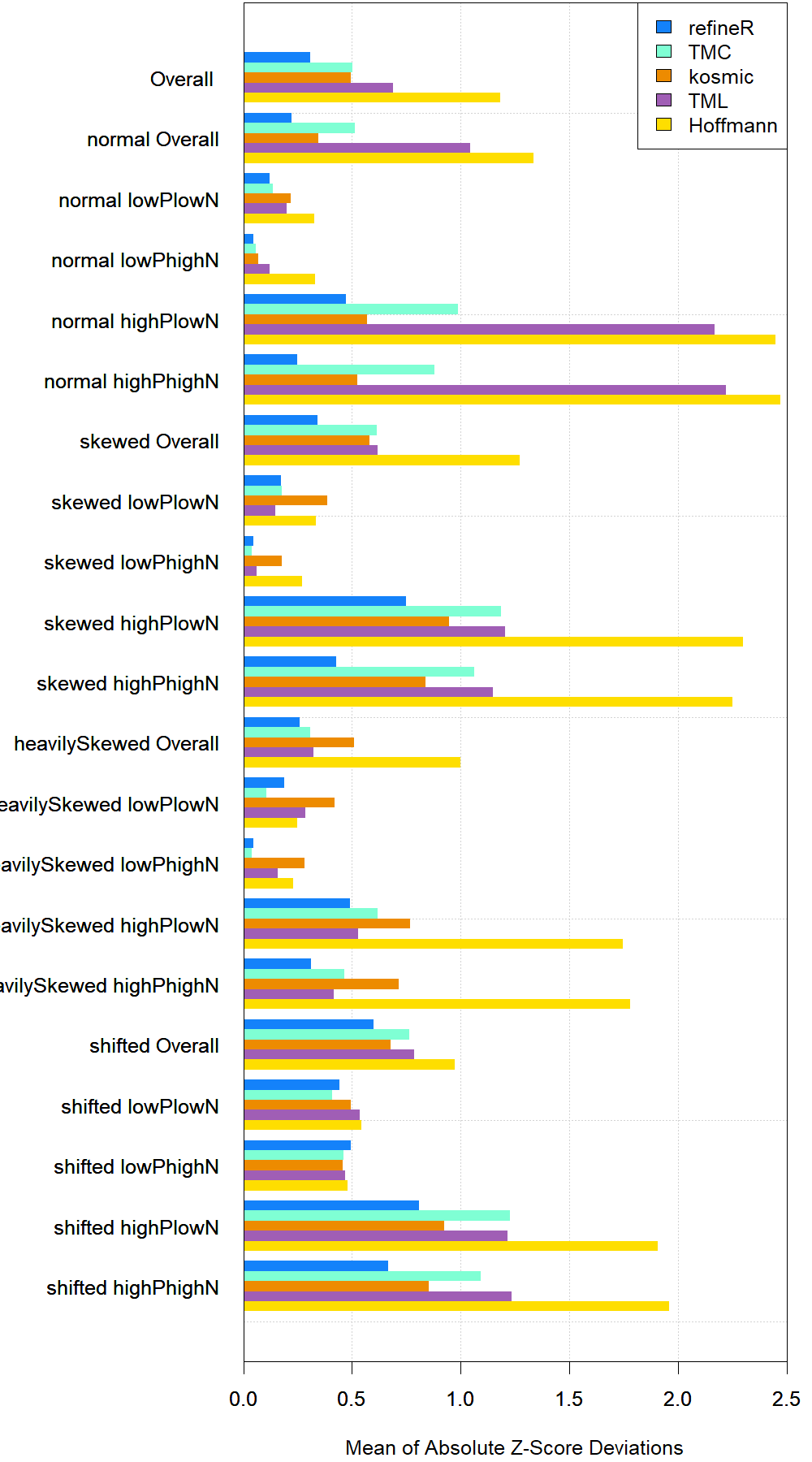Barplot of mean absolute z-score deiviation for the whole set and pre-defined subsets.

Examples for boxplots split by distribution type: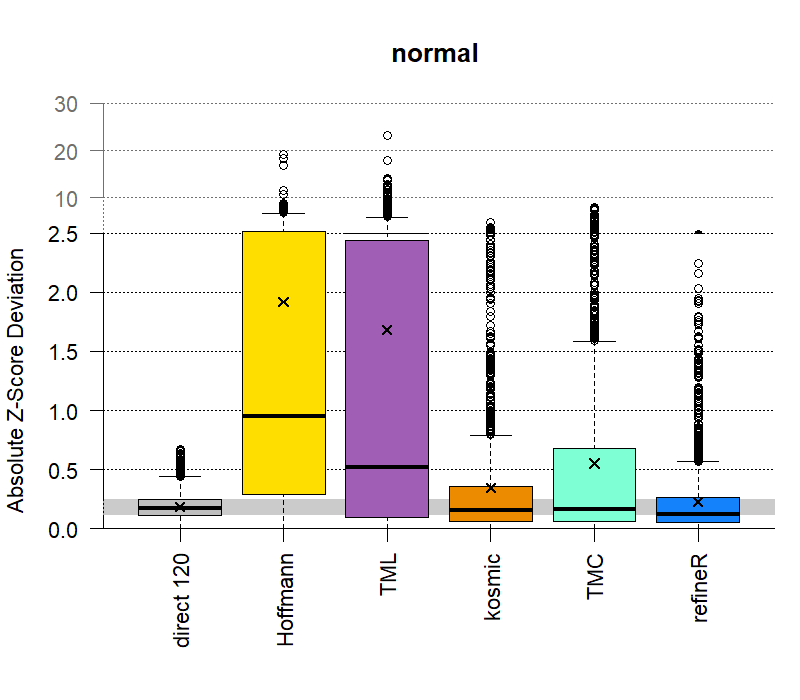Performance comparison for normal distributions.

Examples for boxplots split by pathological fraction or sample size for a certain distribution type: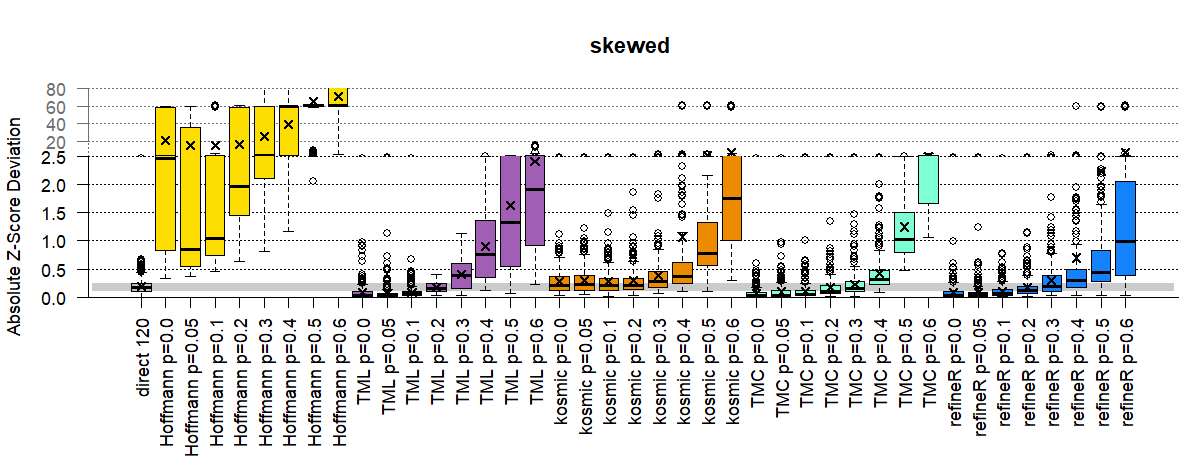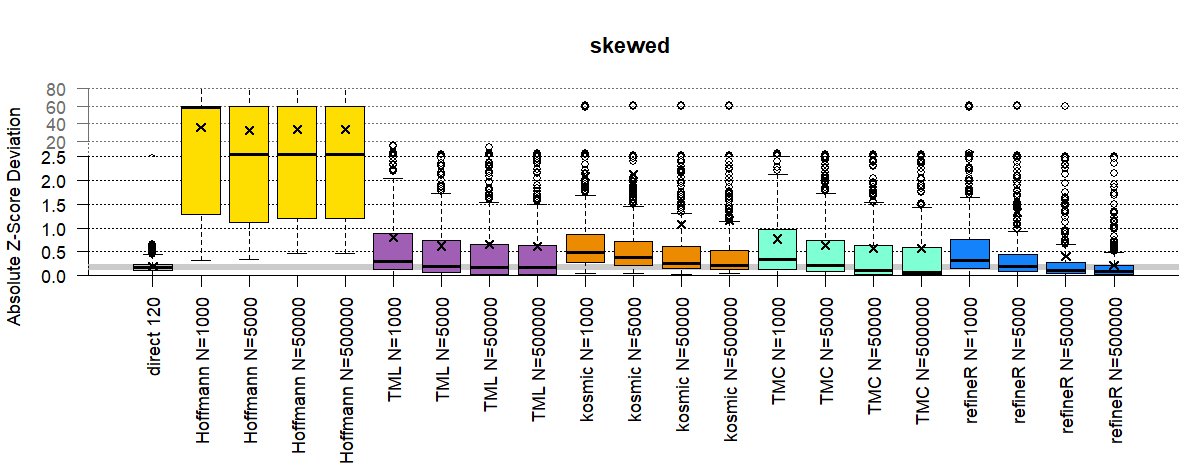To just evaluate the results for one algorithm, e.g. refineR, just set algoNames to the name of the respective method:

# define color for refineR
col_refineR <- rgb(20, 130, 250, maxColorValue = 255)
# evaluate results for only one algorithm
benchmarkScore <- evaluateAlgorithmResults(workingDir = workingDir, algoNames = "refineR", cols = col_refineR)
Exemplary plots created for the evaluation of one algorithm, refineR.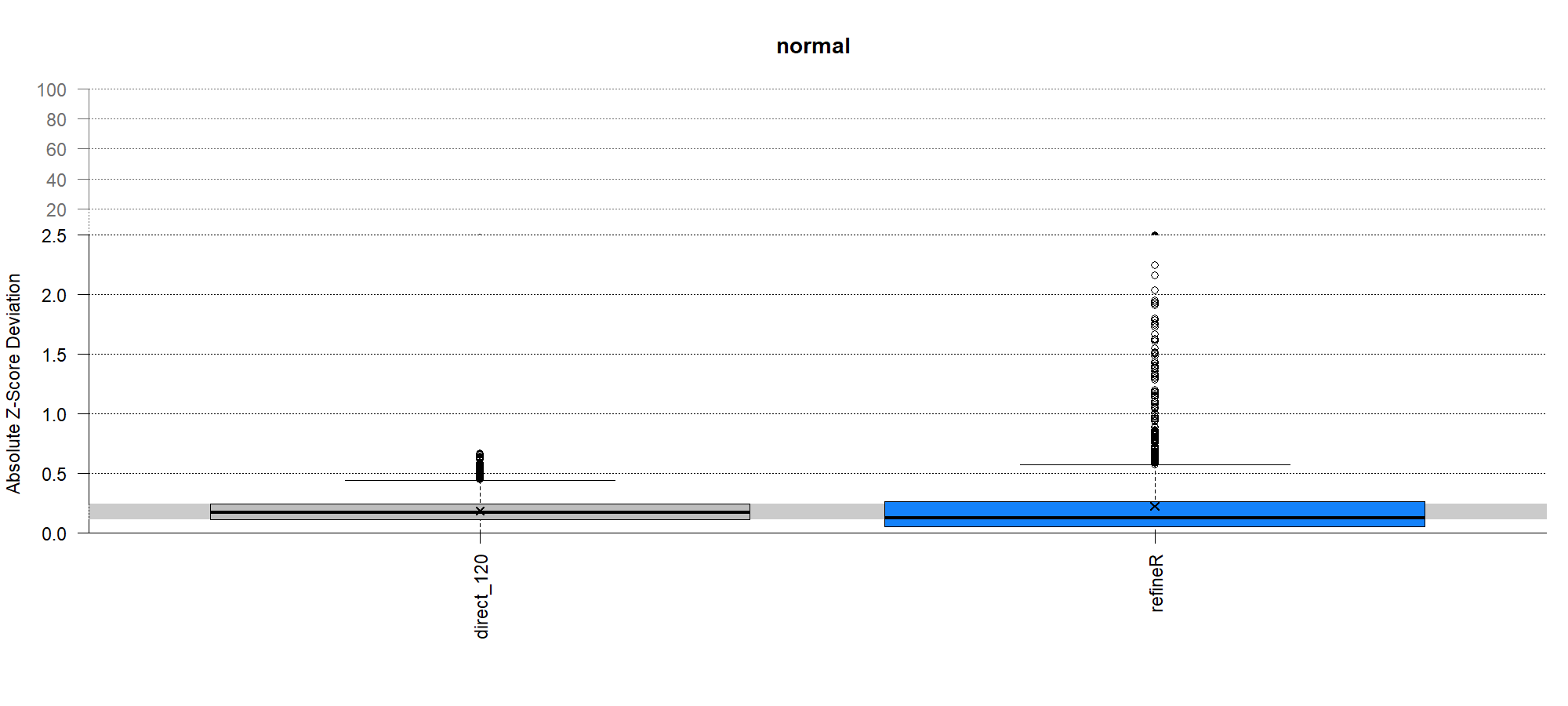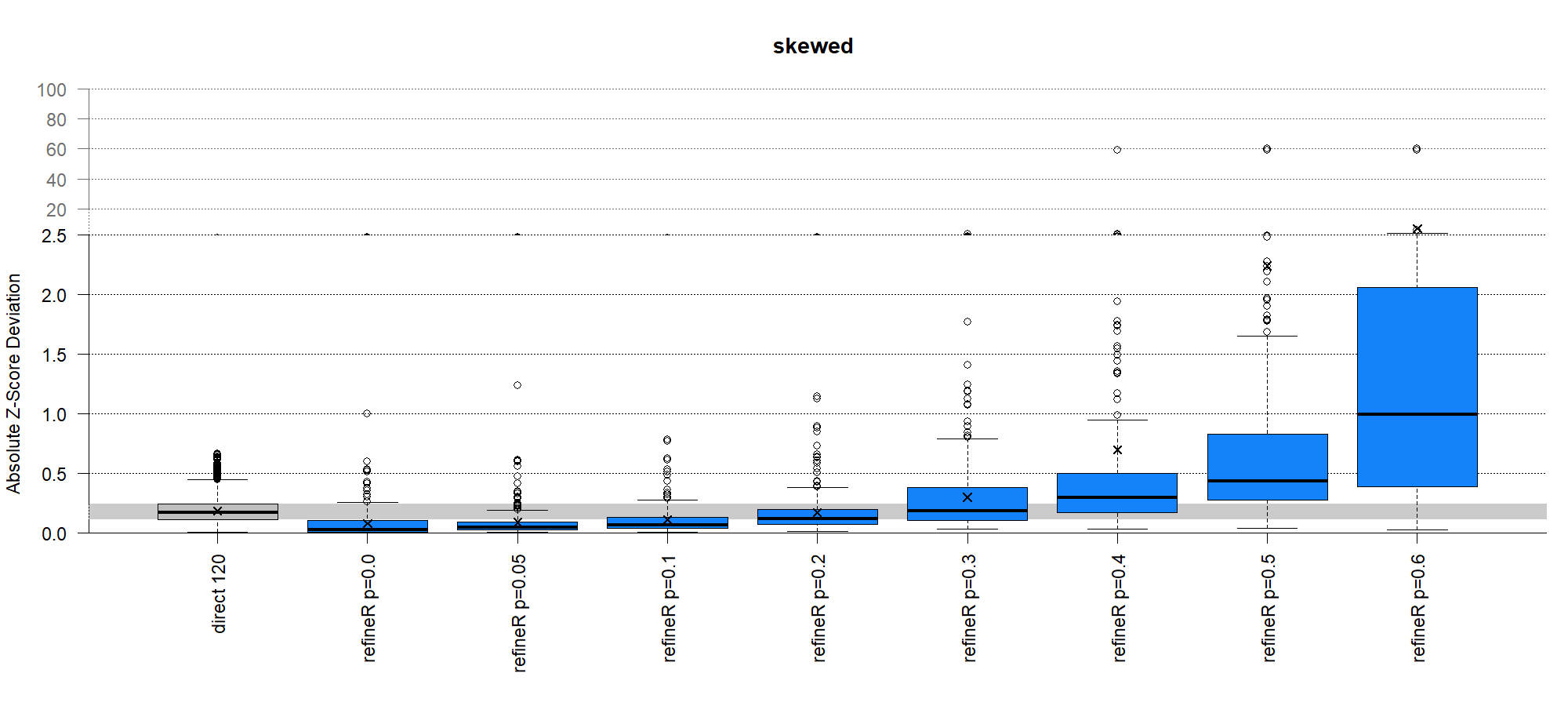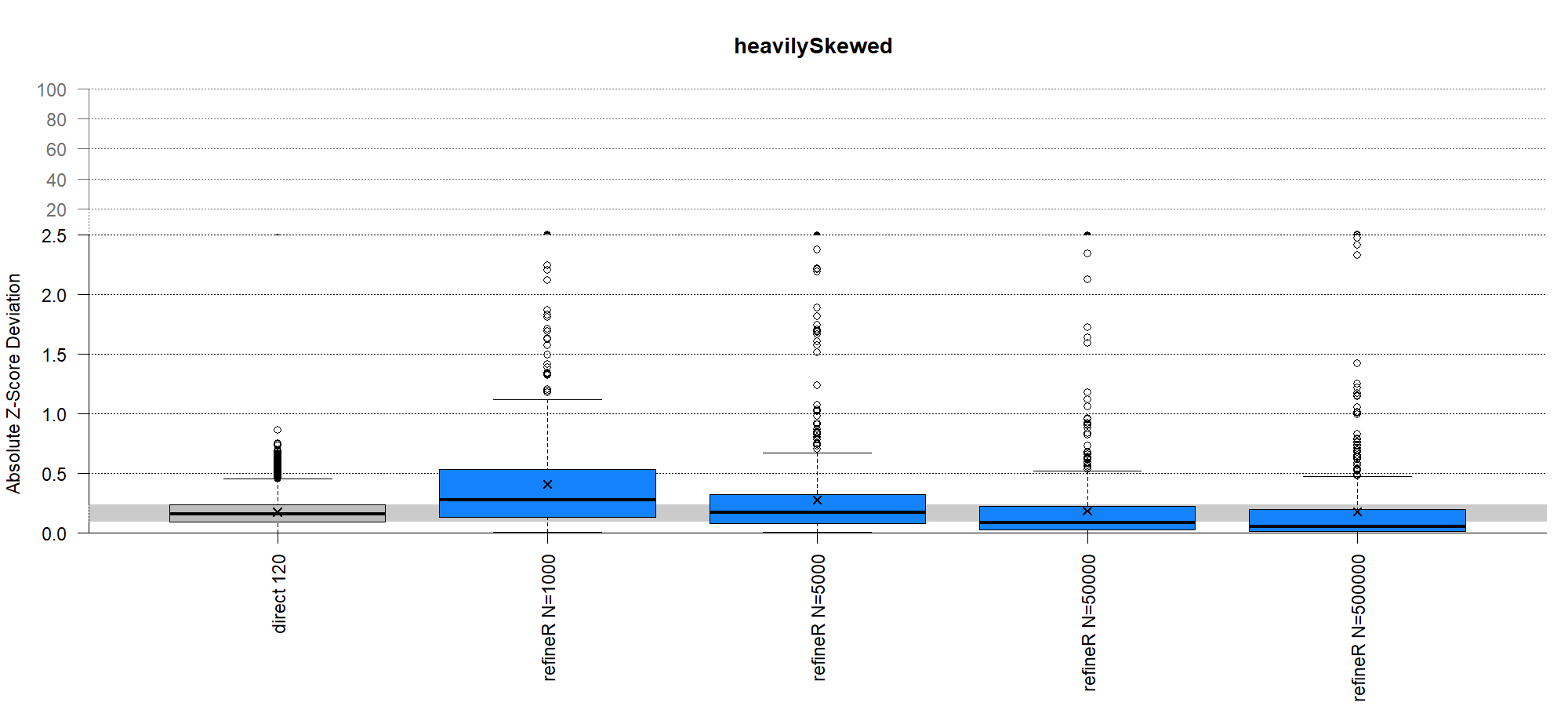### Custom Options

Additionally, plots can be generated for different subsets, similar to generating and evaluating the test sets, e.g. for just one biomarker (“Ca”), one distribution type (“skewed”), or a customized subset.

# define color for TML
col_TML     <- rgb(160,94,181,maxColorValue =255)

# evaluate results for only one biomarker and set color
benchmarkScore <- evaluateAlgorithmResults(workingDir = workingDir, algoNames = "TML", subset = 'Ca', cols = col_TML)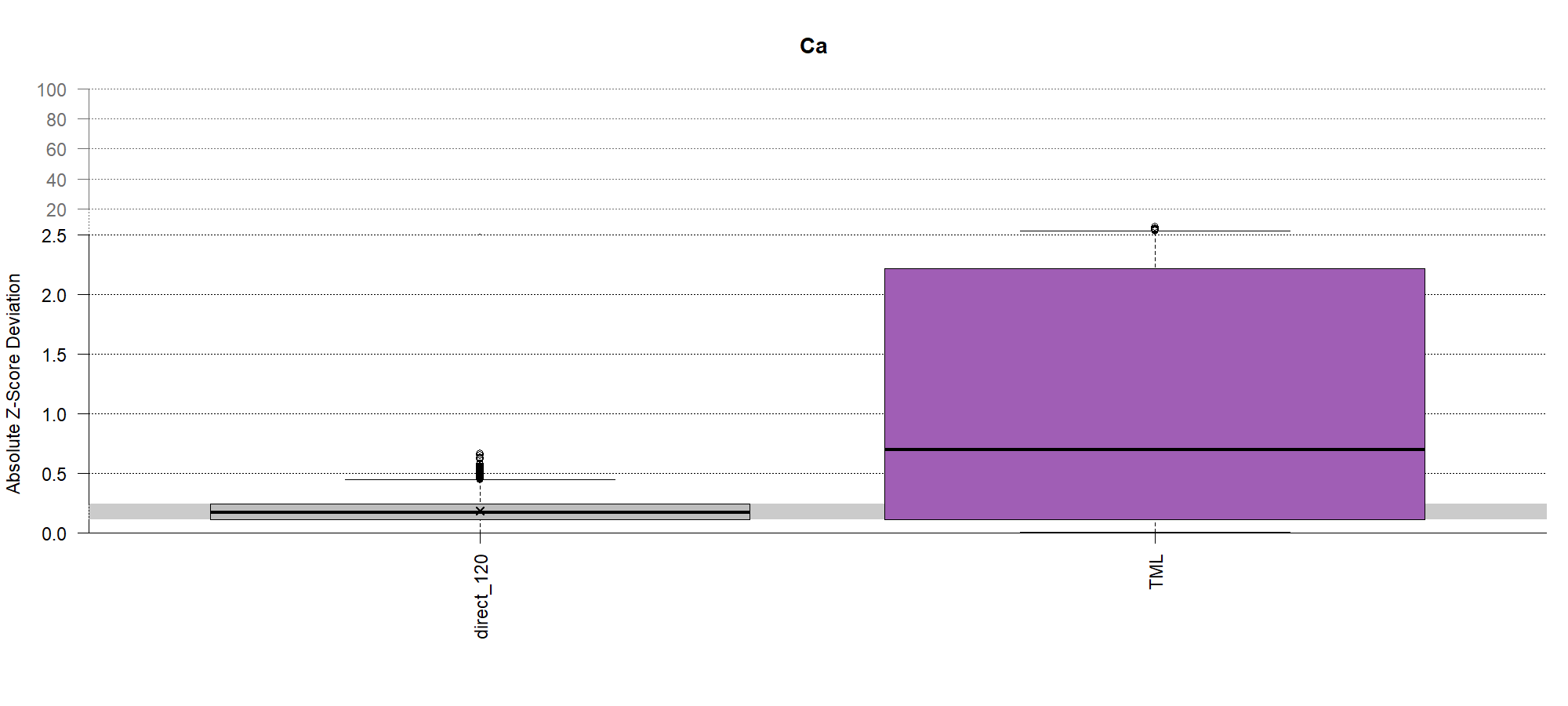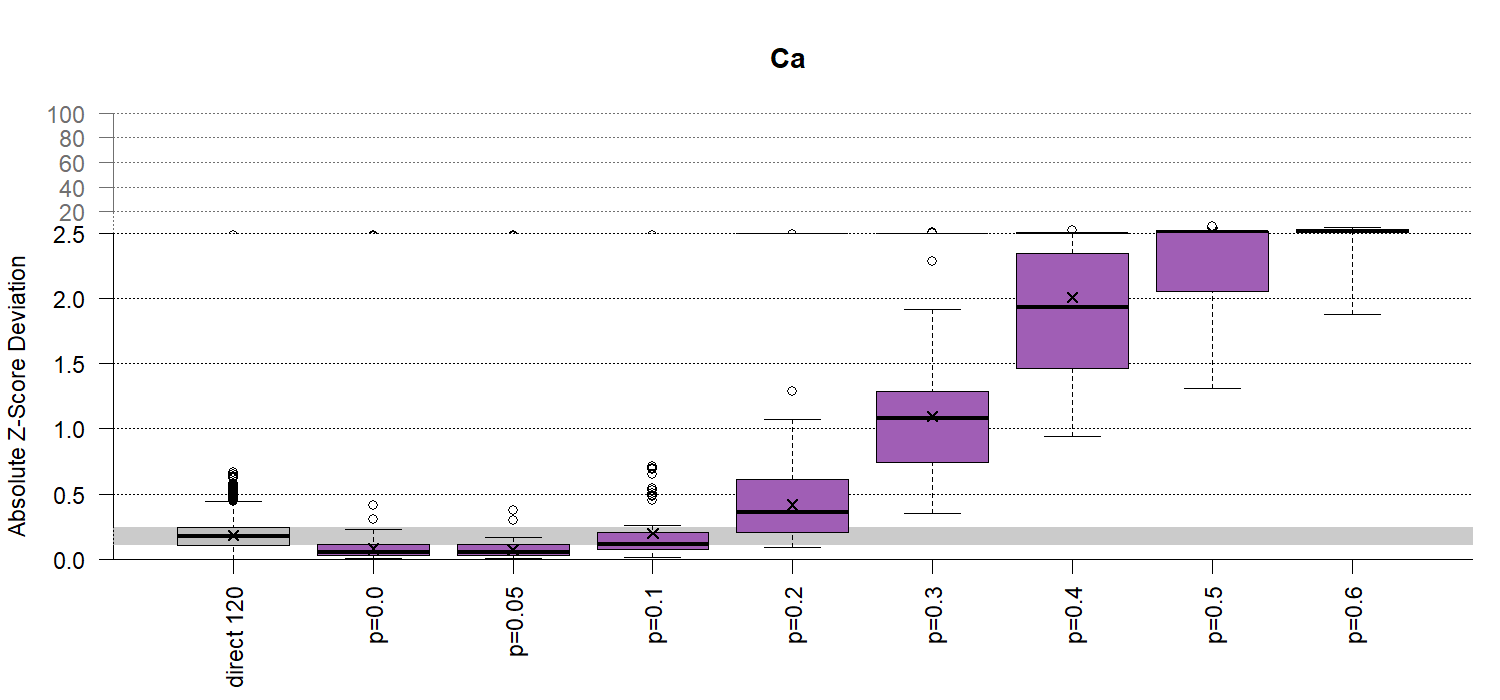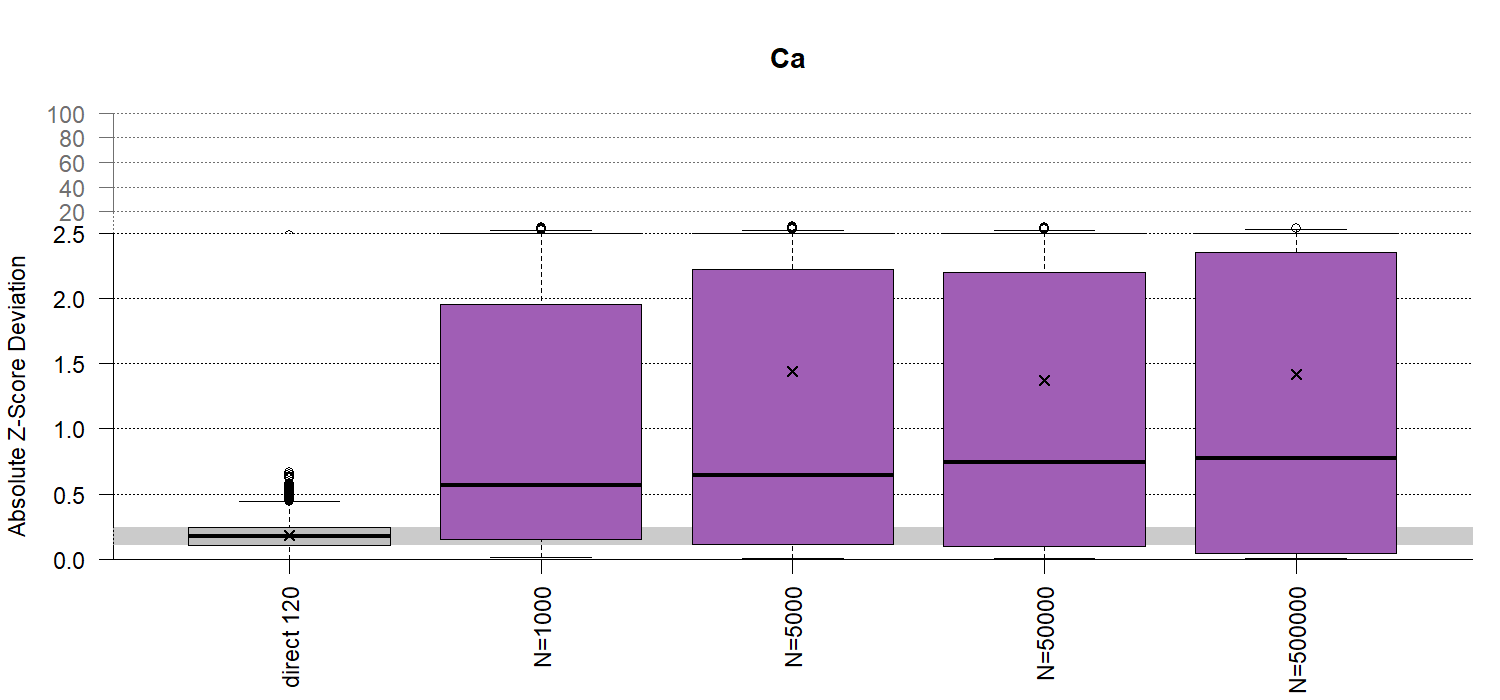# define color for TMC
col_TMC     <- rgb(127, 255, 212, maxColorValue = 255)

# evaluate results for only one distribution type
benchmarkScore <- evaluateAlgorithmResults(workingDir = workingDir, algoNames = "TMC", subset = 'skewed', cols = col_TMC)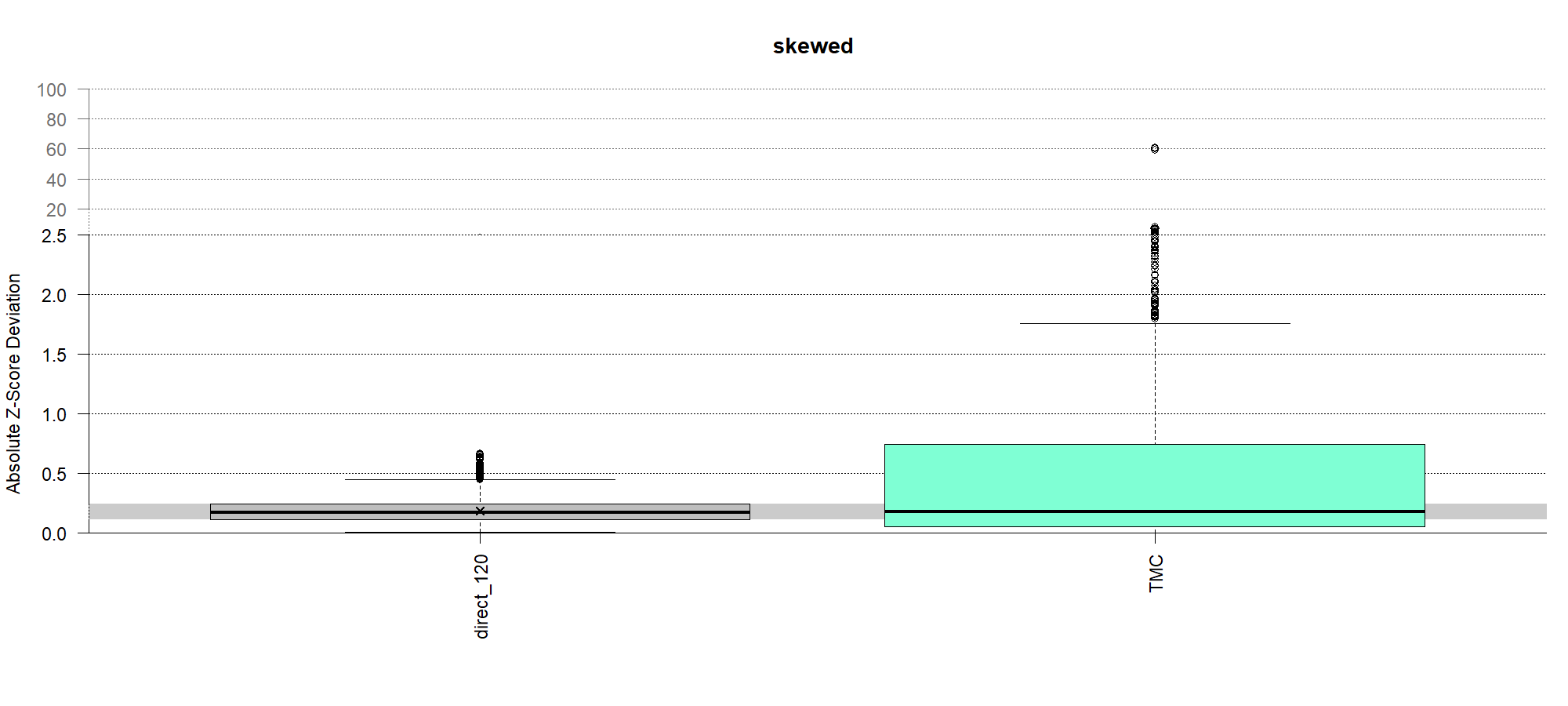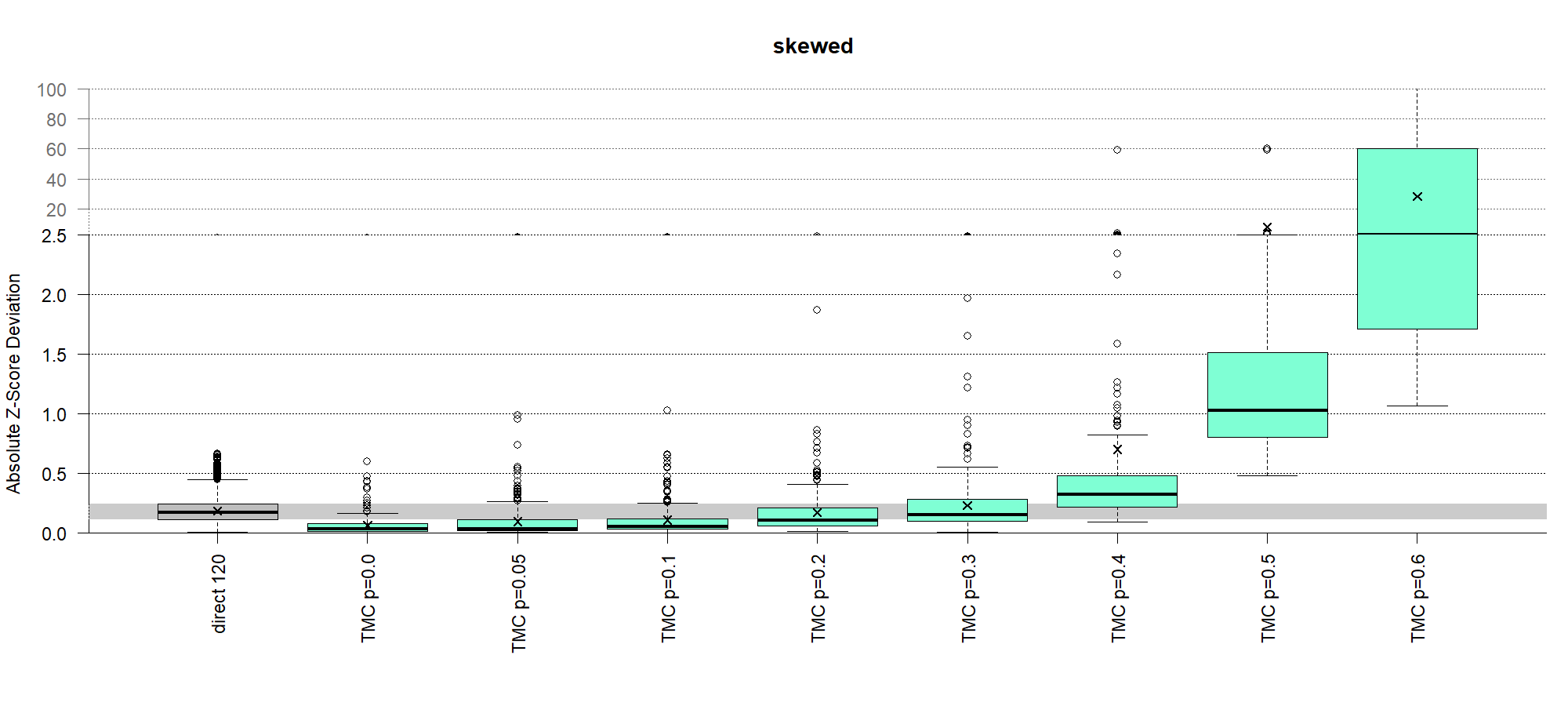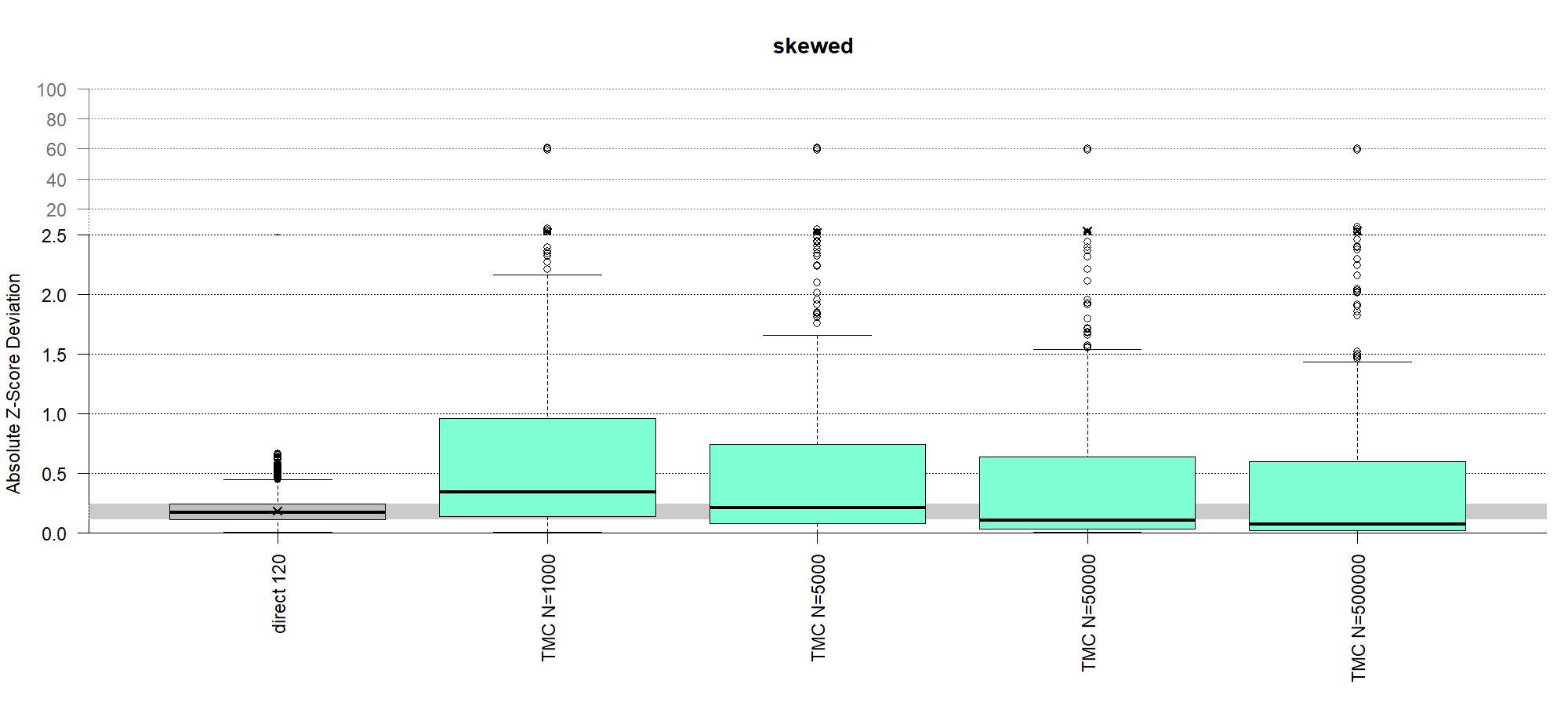# define color for kosmic
col_kosmic  <- rgb(237,139,0,maxColorValue =255)
# evaluate results for only a subset of testsets with defined characteristics (i.e. pathological fraction <= 30%)
benchmarkScore <- evaluateAlgorithmResults(workingDir = workingDir, algoNames = "refineR",
subset = testsets[testsets\$fractionPathol <= 0.3,], cols = col_kosmic)
Example plots for custom subset evaluation for normal distributions: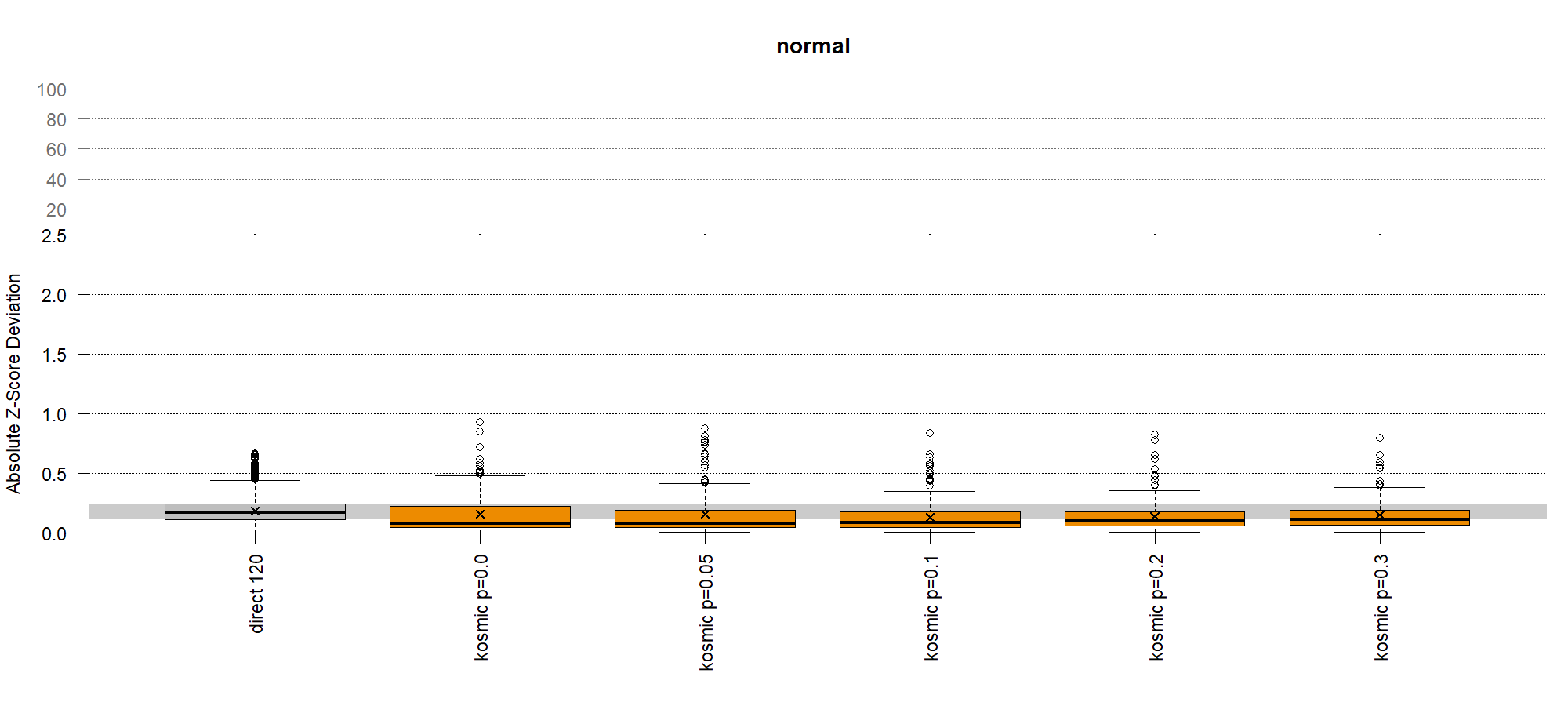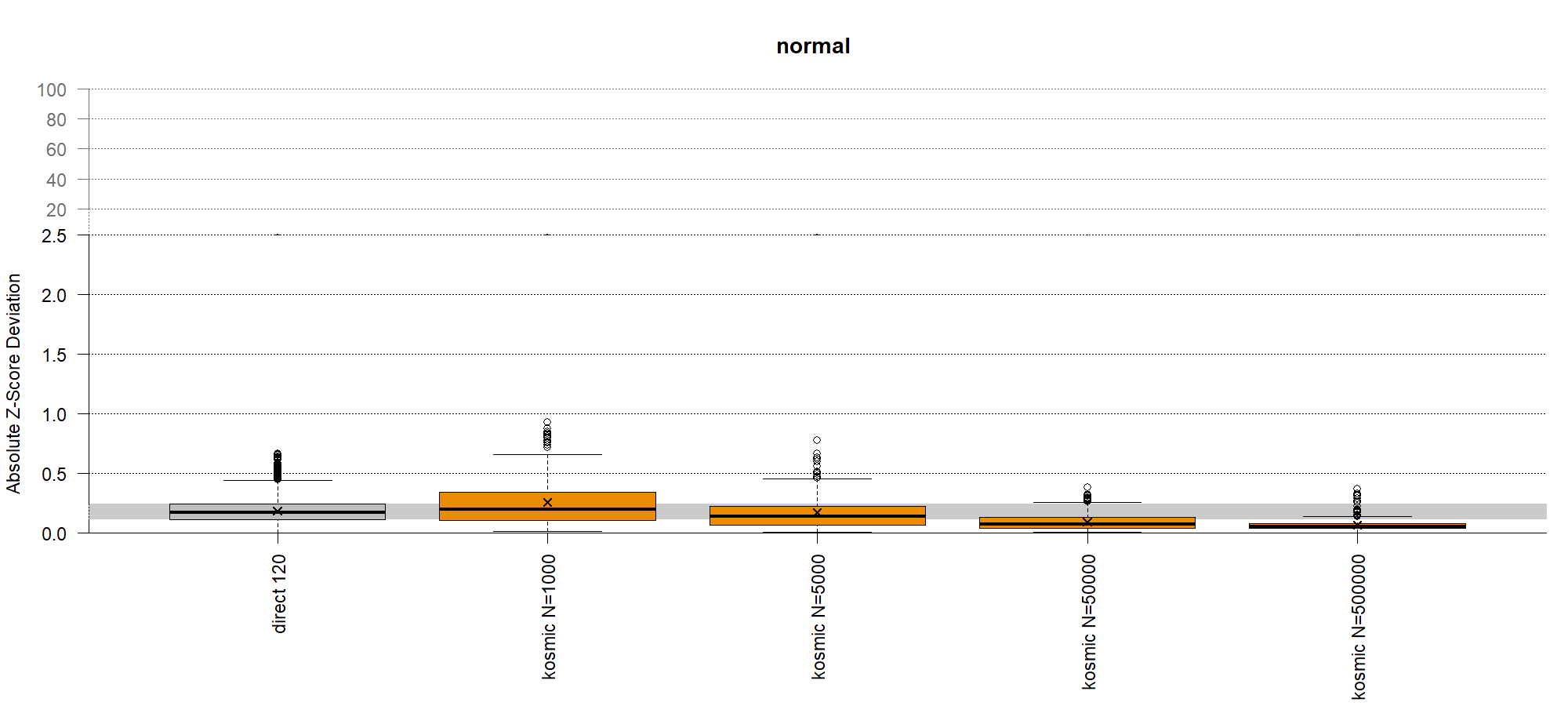## Example: Application of RIbench with refineR

To provide a working example, we apply the refineR algorithm to the benchmark suite, as the package is easy to integrate and we are most familiar with this method.

# load RIbench package
library(RIbench)
# set directory from where a ./Data folder will be generated
workingDir <- tempdir()

# generate all test sets
generateBiomarkerTestSets(workingDir = workingDir, subset = 3)

# evaluate all test sets using existing or new indirect method ('myOwnAlgo') with pre-specified R-function ('estimateModel')
evaluateBiomarkerTestSets(workingDir = workingDir, algoName = 'refineR', algoFunction = 'findRI', libs = c('refineR'), subset = 3)

# evaluate all results, create plots and compute the benchmark score
benchmarkScore <- evaluateAlgorithmResults(workingDir = workingDir, algoNames = "refineR", subset = 3)